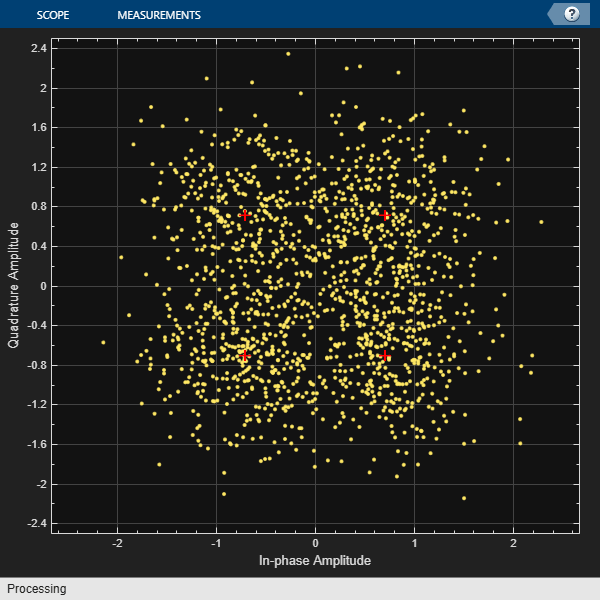# lteConvolutionalDecode

Convolutional decoding

## Syntax

``out = lteConvolutionalDecode(softBits)``

## Description

example

````out = lteConvolutionalDecode(softBits)` returns `out`, data recovered by convolutionally decoding `softBits`, a vector of soft bits.The decoder uses a soft input wrap-around Viterbi algorithm without any quantization. The algorithm creates training data to append to the start and end of the packet by cyclically extending the packet. The traceback decoding length is 42.```

## Examples

collapse all

Generate random bits and convolutionally encode them.

```txBits = randi([0 1],1000,1); codedData = lteConvolutionalEncode(txBits);```

QPSK modulate the coded bits and add noise to the received symbols.

```txSym = lteSymbolModulate(codedData,'QPSK'); noise = 0.5*complex(randn(size(txSym)),randn(size(txSym))); rxSym = txSym + noise;```

Show `rxSymbols` constellation, setting `txSymbols` as the reference constellation.

```xylimits = [-2.5 2.5]; cdScope = comm.ConstellationDiagram('ReferenceConstellation',txSym,'XLimits',xylimits ,'YLimits',xylimits); cdScope(rxSym)```Demodulate the noisy symbols to obtain soft bits, convolutionally decode the soft bits, and display the number of erroneous bits.

```softBits = lteSymbolDemodulate(rxSym,'QPSK','Soft'); out = lteConvolutionalDecode(softBits); disp(sum(out ~= int8(txBits)))```
``` 0 ```

## Input Arguments

collapse all

Soft bit data, specified as a column vector. The function assumes that input data has been encoded by a tail-biting convolutional code with constraint length 7, coding rate 1/3, and octal polynomials G0 = 133, G1 = 171 and G2 = 165. The function also assumes that the input data vector is structured as three encoded parity streams concatenated block-wise in the form `[D0 D1 D2]`, where `D0`, `D1`, and `D2` are the separate parity streams resulting from the original encoding with individual polynomials G0, G1, and G2.

Data Types: `double`

## Output Arguments

collapse all

Convolutionally decoded data, returned as a column vector. The length of this vector is 1/3 of the length of the `softBits` input.

Data Types: `int8`

## Version History

Introduced in R2014a